# Precalculus : Find the Sum and Product of the Zeros of a Polynomial

## Example Questions

### Example Question #1 : Find The Sum And Product Of The Zeros Of A Polynomial

Given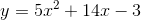, determine the sum and product of the zeros respectively.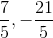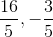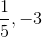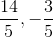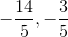Explanation:

To determine zeros of, factorize the polynomial.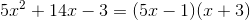Set each of the factorized components equal to zero and solve for.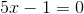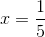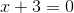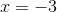The sum of the roots: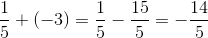The product of the roots: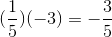### Example Question #1 : Find The Sum And Product Of The Zeros Of A Polynomial

Find the sum and product of the zeros of the following polynomial: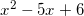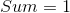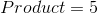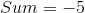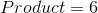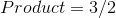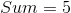Explanation:

To find the zeros you have to factor the polynomial.

This is easily factorable and you will get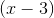and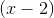.

Next, set both of these equal to zero.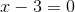and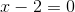.

Isolate the x's and you will getand.

The sum will besince you add the two together, and the product will bebecause you multiply the two together.

### Example Question #2 : Find The Sum And Product Of The Zeros Of A Polynomial

Find the sum and product of the zeros of the following polynomial: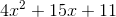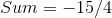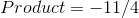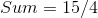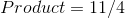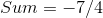Explanation:

Factoring the polynomial will give you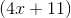and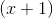.

The zeros of these binomials will be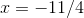and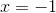.

If you add these together (sum) you get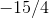and if you multiply them together (product) you get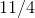.

### All Precalculus Resources